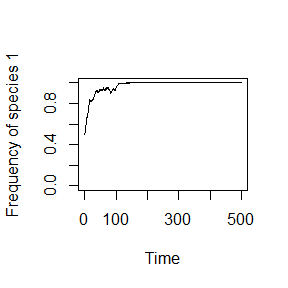## Online Box 6: R code to simulate a colonization-competition tradeoff model in 2 patches: Figure 6.10 in the book

init.1 & m can be set to reproduce each panel in Figure 6.10

WARNING: This code is slower to run than the code in Boxes 1-5 (~40 seconds)

# specify parameters, initial conditions, and output matrix
num.years <- 500
num.patch <- 2
freq.1.mat <- matrix(nrow = num.years, ncol = num.patch)

J <- 1000
init.1 <- 0.5*J
COM <- matrix(nrow=J, ncol=num.patch)
COM[1:init.1,] <- 1; COM[(init.1+1):J,] <- 2

year <- 2

m <- 0.05
fit.ratio.avg <- vector(length=num.patch)
fit.ratio.avg[] <- c(1.2,1.2)
fit.ratio.m <- 1/5
freq.dep <- vector(length=num.patch)
freq.dep[] <- 0

## record data (frequency of species 1) for year 1
freq.1.mat[1,] <- init.1/J

## run simulation
for (i in 1:(J*num.patch*(num.years-1))) {

## choose a patch where a death even will occur
patch <- sample(1:num.patch,1)

## calculate Pr.1 if dispersal occurs
if (runif(1) < m) {
freq.1.meta <- sum(COM==1)/(J*num.patch)
Pr.1 <- fit.ratio.m*freq.1.meta/(fit.ratio.m*freq.1.meta + (1-freq.1.meta))
} else {

## calculate Pr.1 if local reproduction (not dispersal)
freq.1 <- sum(COM[,patch]==1)/J; freq.2 <- 1 - freq.1
fit.ratio <- exp(freq.dep[patch]*(freq.1-0.5) + log(fit.ratio.avg[patch]))
Pr.1 <-  fit.ratio*freq.1/(fit.ratio*freq.1 + freq.2)
}

COM[ceiling(J*runif(1)),patch] <- sample(c(1,2), 1, prob=c(Pr.1,1-Pr.1))

## record data
if (i %% (J*num.patch) == 0) {
freq.1.mat[year,] <- colSums(COM==1)/J
year <- year + 1
}
}

## graph the results
plot(1:num.years, rowMeans(freq.1.mat), type="l", xlab="Time",
ylab="Frequency of species 1", ylim=c(0,1))### Description of the code.

1. As with other multi-patch models, we define fit.ratio.avg and freq.dep as vectors, with one value for each patch. Differences in dispersal ability are represented by fit.ratio.m, which is used to calculate the probability that - in the event of dispersal - the reproducing individual is of one species or the other. If fit.ratio.m > 1, species 1 is a better disperser and vice versa.
m <- 0.05
fit.ratio.avg <- vector(length=num.patch)
fit.ratio.avg[] <- c(1.2,1.2)
fit.ratio.m <- 1/5
freq.dep <- vector(length=num.patch)
freq.dep[] <- 0
1. With probability m the reproducing individual will be chosen from the entire metacommunity, but the choice is not random if fit.ratio.m ≠ 1. freq.1.meta is the frequency of species 1 in the metacommunity, and then fit.ratio.m is used to calculate Pr.1 in the exact same way as fit.ratio is used for selection occurring within a local community.
  if (runif(1) < m) {
freq.1.meta <- sum(COM==1)/(J*num.patch)
Pr.1 <- fit.ratio.m*freq.1.meta/(fit.ratio.m*freq.1.meta + (1-freq.1.meta))
} else { 
1. Unlike the case in Boxes 1-5, here we plot the average frequency across patches, instead of the frequency within one or each patch. Mean frequency of species 1 is calculated by rowMeans(freq.1.mat)
plot(1:num.years, rowMeans(freq.1.mat), type="l", xlab="Time",
ylab="Frequency of species 1", ylim=c(0,1))